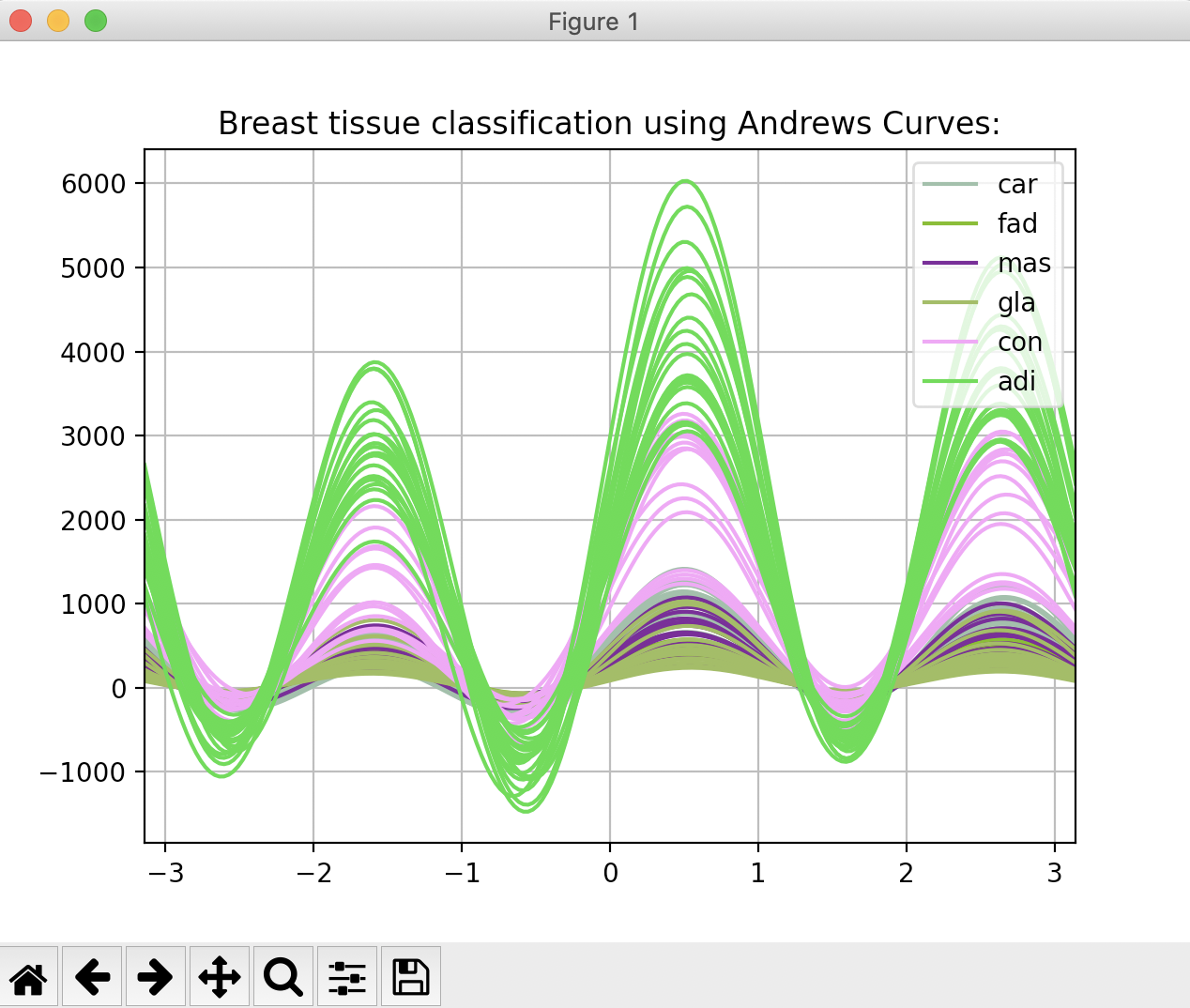# Drawing Andrews Curves Using Pandas

## Overview:

• Andrews curves project multivariate data, through the vector formed by the co-efficients of the finite Fourier series on to a two-dimensional space.
• The Fourier series and the vector is given by
1. x(t) = x1/√2 +x2sin(t) +x3cos(t) +x4sin(2t) +x5cos(2t)
2. 1/√2,sin(t),cos(t),sin(2t),cos(2t), . .
• Each data point "t" in the multivariate data, is represented by a curve between −π and π.
• If two curves appear closer in the Andrews Curves plot, then these two data points are indeed closer.
• Given a "class" column of a pandas DataFrame, the function andrews_curves() from the plotting module of the pandas library, draws Andrews curves.

## Example 1:

 # Example Python program that plots # Andrews curves for a multivariate data # Data Courtesy: https://archive.ics.uci.edu/ml/datasets/Breast+Tissue import pandas as pds import matplotlib.pyplot as plt # Read data from a CSV file into a DataFrame df = pds.read_csv("BreastTissue.csv"); # Draw Andrews curves pds.plotting.andrews_curves(df, "Class"); plt.title("Breast tissue classification using Andrews Curves:"); plt.show(block=True);

## Output: